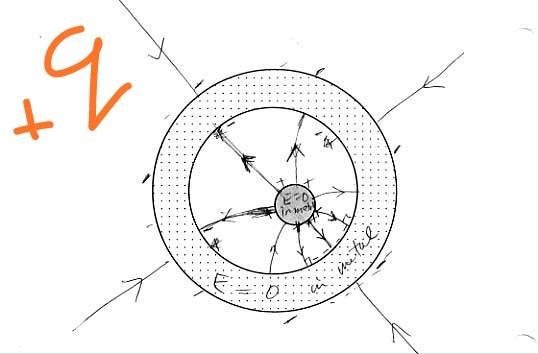# Electrostatic Shielding

• navneet9431

#### navneet9431

Gold MemberWhat is true is that the field due to the point charge outside of the conductor will not be able to penetrate the shell i.e. there will be no field due to the external point charge anywhere within the conductor nor in the cavity: the field will be **killed off*& by the charges on the outer surface of the conductor. Similarly, the field due to the point charge in the cavity will also vanish for all points exterior to the cavity because it will be **killed off** by the charges on the inner surface of the conductor.

Can you please explain this phenomenon of killing of electric field lines more clearly?

I will be thankful for help!

What would happen with the charge carriers if there would be an ##\vec E## field left over in the conductor ?
Similarly, the field due to the point charge in the cavity will also vanish for all points exterior to the cavity
This is not correct -- only true for points inside the conductor!

What would happen with the charge carriers if there would be an ##\vec E## field left over in the conductor ?
This is not correct -- only true for points inside the conductor!
Suppose,we have a solid spherical conductor with a cavity in it and a charge +q placed outside the sphere.Then how is the electric field inside the cavity zero?Can you please explain this?

Are you aware of the Gauss theorem ?

The charge carriers in the conductor will redistribute on the outside of the conductor in such a way that the ##\vec E\;## field lines are all perpendicular to the outer surface.

In the conductor itself there is no ##\vec E\;## field and on the inner surface the mirror charge is evenly distributed (can you see why ?) so there is no field inside the cavity.

Are you aware of the Gauss theorem ?

The charge carriers in the conductor will redistribute on the outside of the conductor in such a way that the ##\vec E\;## field lines are all perpendicular to the outer surface.

In the conductor itself there is no ##\vec E\;## field and on the inner surface the mirror charge is evenly distributed (can you see why ?) so there is no field inside the cavity.
Ok thanks!
I can see things getting clear..
But,what actually prevents the electric field lines generated by external charge *to penetrate and get inside* the cavity throght the material of the conductor and make the electric field inside the cavity non-zero?
this is my main doubt.

If an isolated conductor without cavities is charged, its excess charge will distribute itself on its surface in order to guarantee that the electric field is zero on its interior.
If instead the conductor had an interior cavity, the charges would again distribute themselves on the outer surface in order to eliminate the electric field on the inside material of the conductor. (Free electrons cannot move in equilibrium.) Why is the electric field within the cavity zero as well?
Or in other words,
what actually prevents the electric field lines generated by external charge *to penetrate and get inside* the cavity throght the material of the conductor and make the electric field inside the cavity non-zero?

Electrostatics with conductors is governed by the equations (in SI units)
$$\vec{\nabla} \times \vec{E}=0, \quad \vec{\nabla} \cdot \vec{E}=\frac{1}{\epsilon_0} \rho.$$
Within the conductors you must have ##\vec{E}=0##, because otherwise there were a current according to Ohm's Law, but electrostatics is defined such that there are no currents.

The first equation tells you that there is a potential
$$\vec{E}=-\vec{\nabla} \Phi.$$
At the boundaries of the conductors the tangential components of the electric field must be continuous and thus vanish, while the normal components jump by the amount ##\sigma/\epsilon_0##, where ##\sigma## is the surface-charge density on the surfaces. This implies that the surfaces of conductors are necessarily equipotential surfaces.

If there are no charges inside your cavities, there you have ##\Delta \Phi=0##, and the surfaces of the cavities are equipotential surfaces. Thus ##\Phi=\text{const}## is a solution inside the cavities, and since the Poisson and Laplace equations have unique solutions under the circumstances here, it's the only solution, but this implies that ##\vec{E}=-\vec{\nabla} \Phi=0## inside the cavities.

,what actually prevents the electric field lines generated by external charge *to penetrate and get inside* the cavity

The mobility of the charge carriers ! (makes sense, since that is the distinguishing difference between a conductor and an insulator)

Can you agree there are no ##\vec E\,## field lines inside the conducting material ? If there were, charge carriers would move, right ?